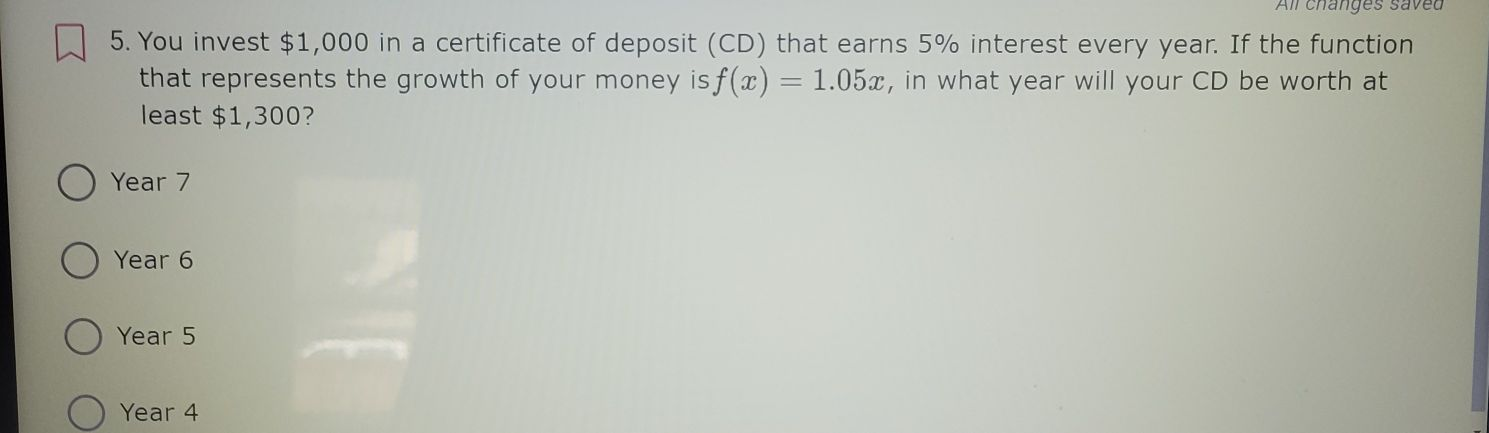### ¿Todavía tienes preguntas de matemáticas?

Pregunte a nuestros tutores expertos
Algebra
Pregunta5. You invest $$\ 1,000$$ in a certificate of deposit $$( C D )$$ that earns $$5 \%$$ interest every year. If the function that represents the growth of your money is $$f ( x ) = 1.05 x$$ , in what year will your CD be worth at least $$\ 1,300$$ ? Year $$7$$ Year $$6$$ Year $$5$$ Year $$4$$

1000×1.05$$^{x}$$=1300